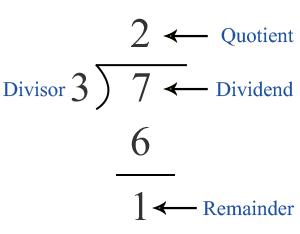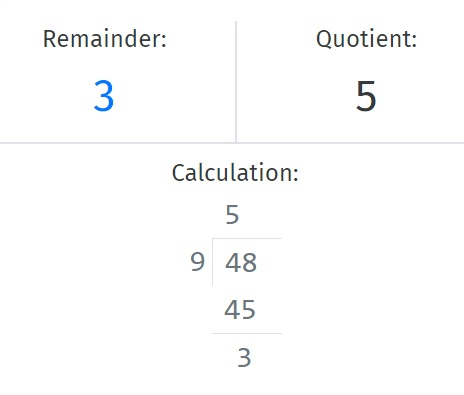# Remainder Calculator

Enter Information#### RESULTS

Fill the calculator form and click on Calculate button to get result here

## Quotient and Remainder Calculator

Remainder calculator is used to calculate the remainder when dividing two digits. It is a remainder theorem calculator that calculates the remainder and quotient in the process of division. When you are working with fractions and using a divisor calculator to divide those fractions, every calculator will give you the result in decimal form. You can use the above remainder and quotient calculator to get a reminder as well as the quotient after the division.

In this post, we will go through the remainder definition, how to use the remainder calculator, and how to find the remainder.

## How to use Remainder Calculator?

The remainder calculator with steps makes the remainder calculation very convenient. You don’t need to know the division process to use this quotient calculator. To make a division with remainders, follow the steps below:

• Enter the dividend in the first input box above.
• Enter the divisor in the next input box labeled as Devisor.
• Press the Calculate button to get the remainder with steps.

This devisor calculator will instantly give you the remainder as well as the quotient after the division. The remainder theorem calculator will also show you the division using a long division method. You can use this calculator with the remainder to get the remainders as well as to learn the division on your own. This remainder finder is very handy for students of mathematics in high school.## How to Find the Remainder?

The remainder can be calculated in multiple ways. You can use our remainder theorem calculator to get quick and accurate results without manual calculation. Here, we will discuss the simplest method to find the remainder. Follow these steps of the quotient calculator to find the remainder of a division.

Step 1: Identify values for the division and specify the numerator (dividend) and denominator (divisor). A numerator or dividend is the number on which the operation of division is performed while a divisor or denominator is the number that divides the dividend. In this case, we will suppose 345 as the numerator and 12 as the denominator. So, the fraction will be:

$$= \dfrac{345}{12}$$

Step 2: Divide the dividend $$(345)$$ with divisor $$(12)$$. You can use your mobile calculator for the division. After dividing, you will get the answer in decimal. In this case, the answer is $$28.75$$. Use our rounding calculator to round the figure to the nearest whole number. After rounding the number, it becomes:

$$= 29$$

Step 3: In this step, multiply the quotient with the denominator or divisor.

$$= 29 \times 12 = 348$$

Step 4: After multiplying, subtract the dividend from the number you get in the previous step.

$$= 348 - 345 = 3$$

After dividing $$345$$ with $$12$$, the remainder is 3You can use the above quotient and remainder calculator to calculate the remainder as you can see, it is only a matter of one click if you use the remainder calculator online.

#### Some other solutions for the remainder calculations

88 divided by 9 => Quotient = 8, Remainder = 7

61 divided by 7 => Quotient = 8, Remainder = 5

88 divided by 12 => Quotient = 7, Remainder = 4

978000 divided by 249 => Quotient = 88909, Remainder = 1

28 divided by 4 equals => Quotient = 7, Remainder = 0

28 divided by 14 => Quotient = 2, Remainder = 0

Other Languages
User Ratings
• Total Reviews 0
• Overall Rating 0/5
• Stars
Reviews
No Review Yet